# 小课堂：如何用Excel制作，财务损益表瀑布图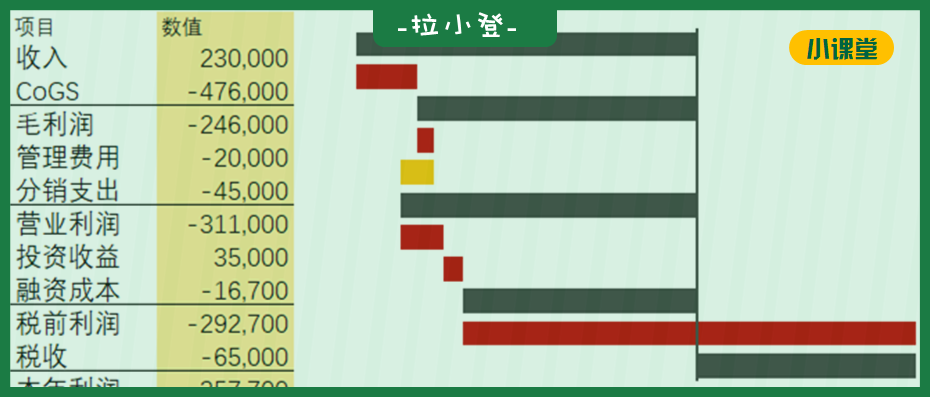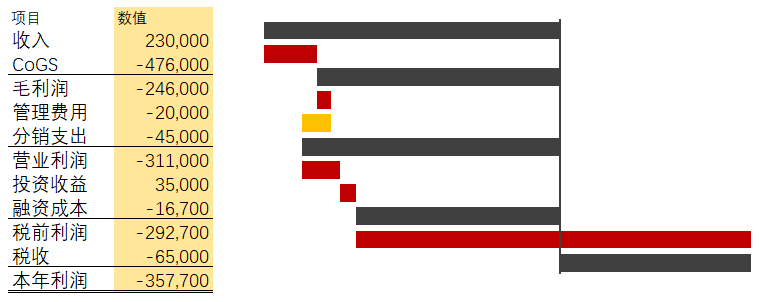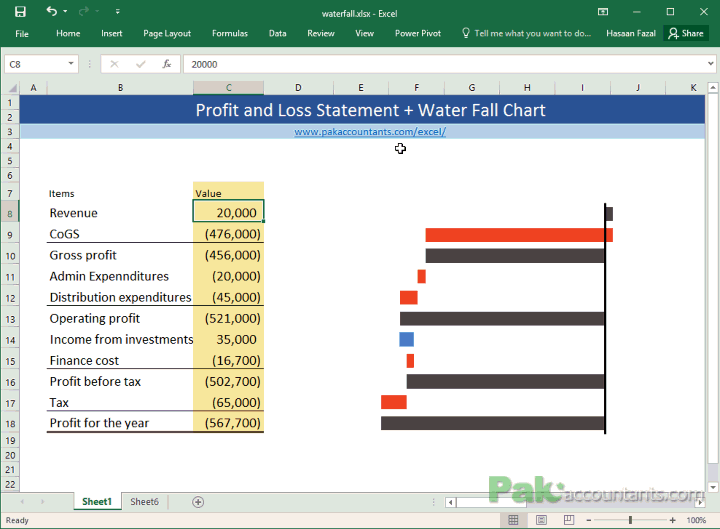# 1- 操作步骤

## 2- 准备辅助列数据

– E7: “Working”

– G7: “Right +ve”

– H7: “Left +ve”

– I7: “Right -ve”

– J7: “Left -ve”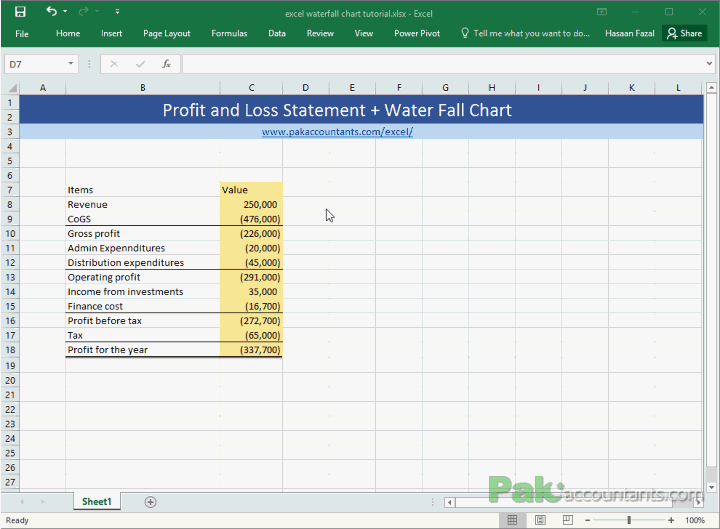## 3- 添加小计公式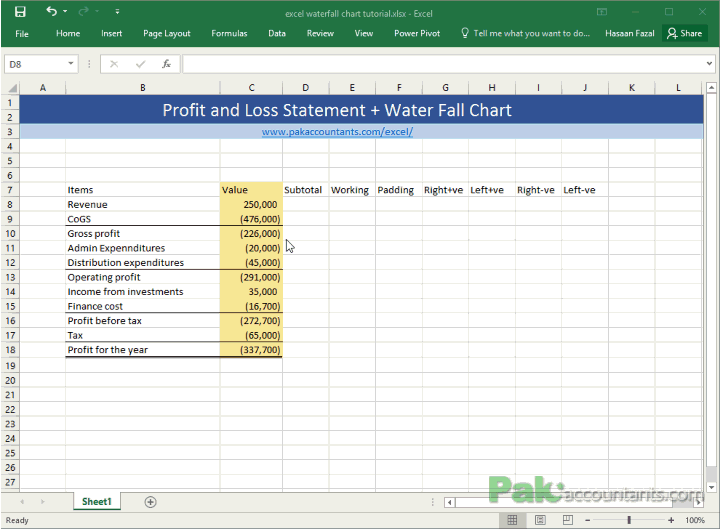## 4- 数据累计求和计算

=IF(D8<>””,D8,SUBTOTAL(9,C\$8:C8))

## 5- 添加Right +ve公式

=IF(ISBLANK(D8),IF(OR(E8<0,C8<0),0,MIN(E8,C8)),0)

## 6- 添加Left +ve公式

=IF(ISBLANK(D8),IF(AND(C8>0,E8<0),-C8,IF(AND(C8>0,E8>0,E8<C8),-(C8-E8),0)),0)

## 7- 添加Right -ve公式

=IF(ISBLANK(D8),IF(AND(C8<0,E8>0),ABS(C8),IF(AND(C8<0,E8<0,E8>C8),ABS(C8-E8),0)),0)

## 8- 添加Left-ve公式

=IF(ISBLANK(D8),IF(E8>0,0,IF(AND(C8<0,E8<0),MAX(C8,E8),0)),0)

=IF(OR(D8<>””,AND(G8<>0,H8<>0),AND(I8<>0,J8<>0)),0,MIN(ABS(E7),ABS(E8))*SIGN(E8))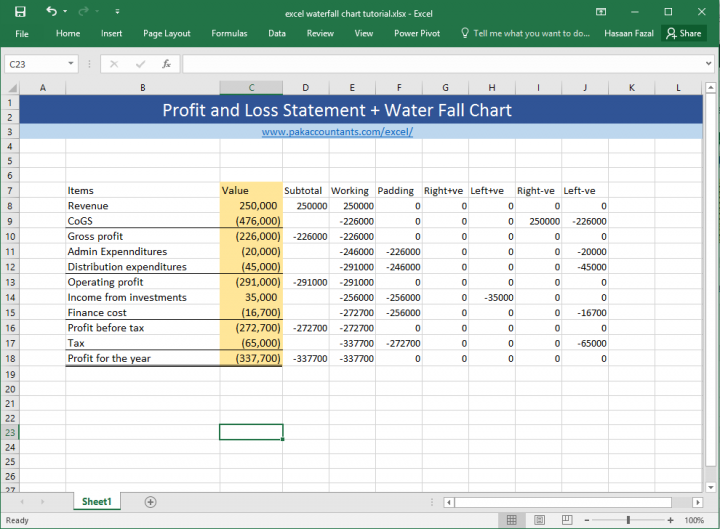## 10- 添加图表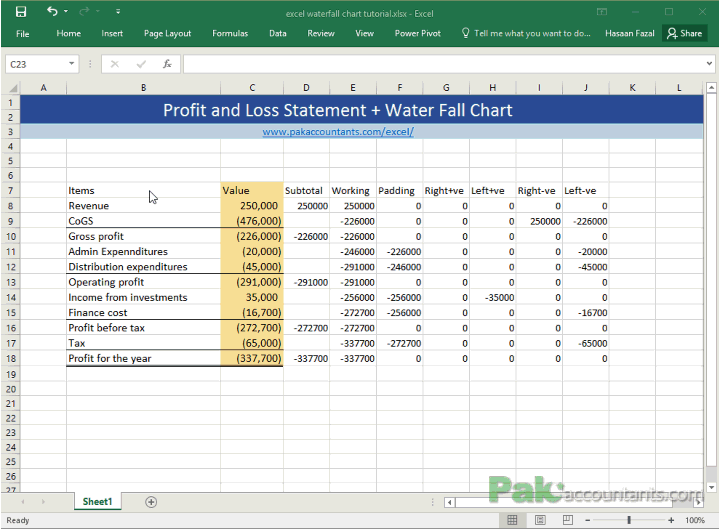## 11- 设置图表属性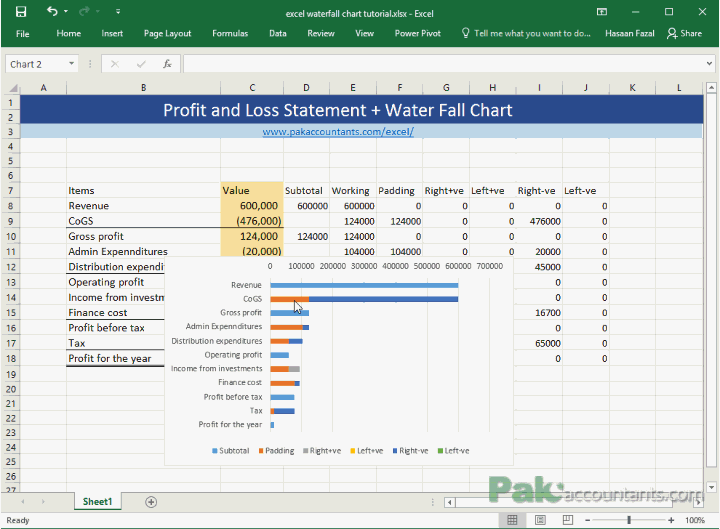## 13- 设置间隙宽度## 14- 删除坐标轴和图例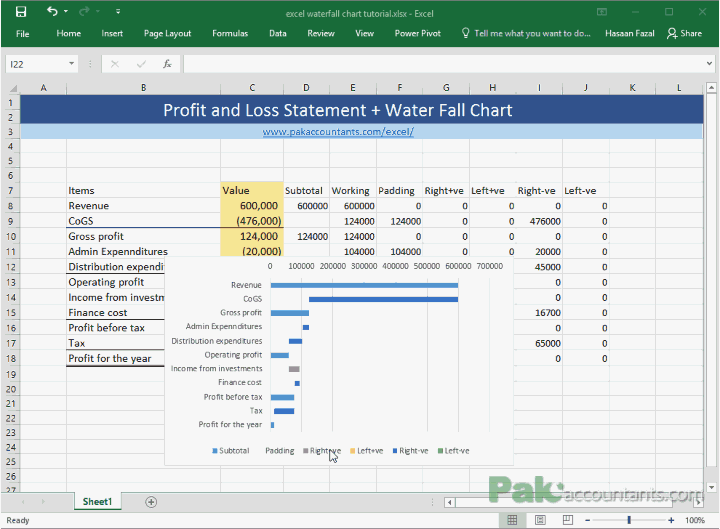## 15- 隐藏y坐标轴标签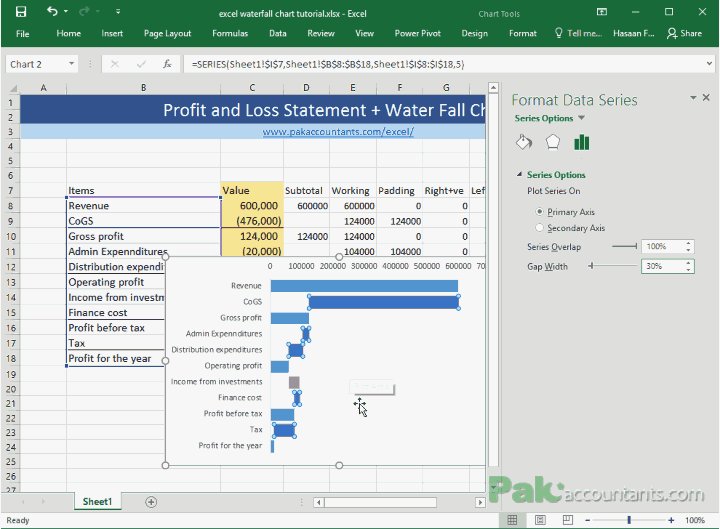## 16- 设置y坐标轴样式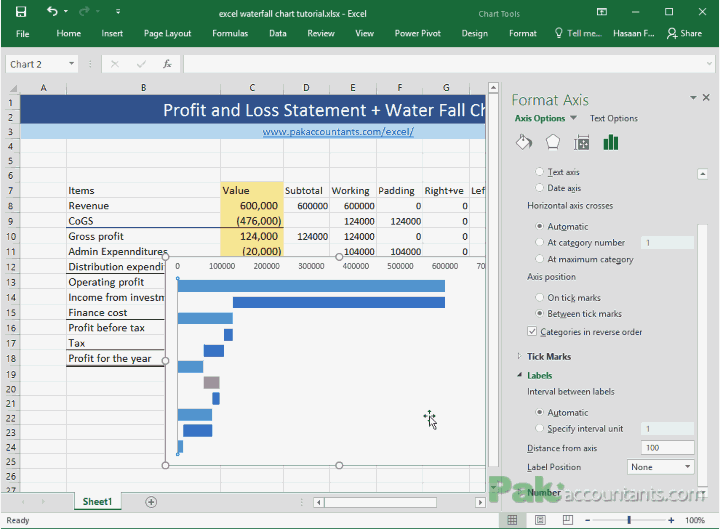## 17- 区分数据系列颜色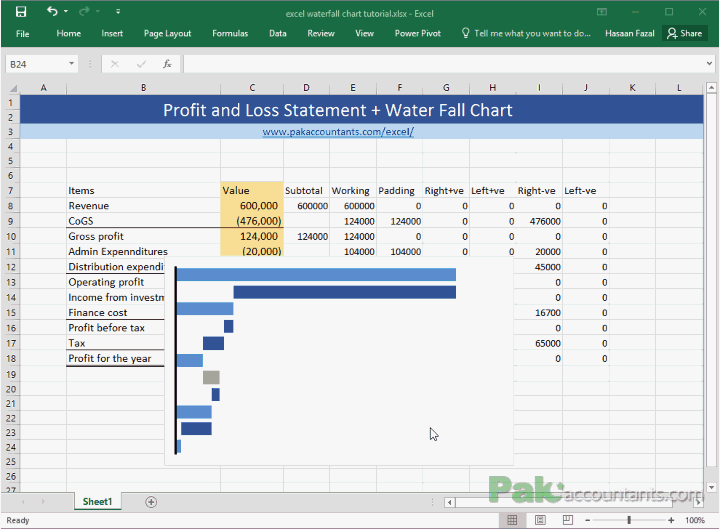## 18- 隐藏图表数据，美化图表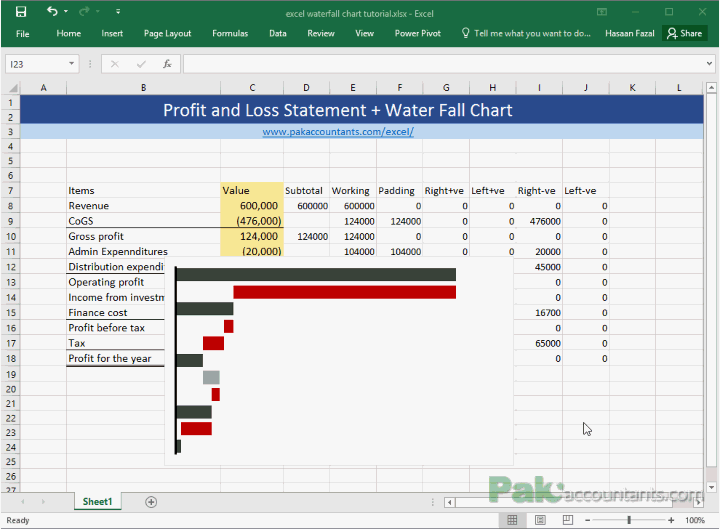# 3- 总结

## 图表学习网站推荐

– 哑铃图

– 损益图

– 甘特图

– 象限图等等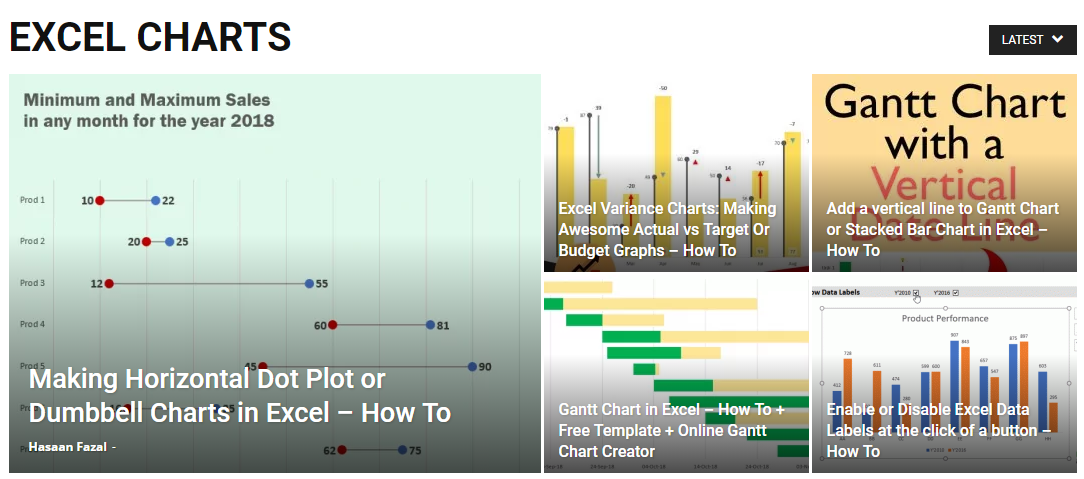### = = 推荐文章 = =

https://pakaccountants.com/category/excel-charts/